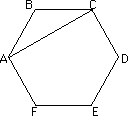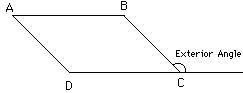Math Central - mathcentral.uregina.caResource Room— glossaries Resource RoomMathematics Glossary - Middle Years Maxine Stinka, Saskatchewan Education, Curriculum and Instruction Note: The definitions included here are those that are used in the Saskatchewan Education document "Mathematics 6-9: A Curriculum Guide for the Middle Level". Various mathematics dictionaries may have different definitions. These definitions are designed to be meaningful to middle level mathematics teachers. Select the first letter of the term that you wish to look for. A | B | C | D | E | F | H | I | J | K | L | M | N | O | P | Q | R | S | T | U | V | W | X | Y | Z D data facts and opinions from which conclusions can be drawn. degree of a: polynomial the greatest of the degrees of its terms. the sum of the number of times each variable appears as a factor. e.g. the degree of the term 5x3y4z is 8 because 3 + 4 + 1 = 8. dependent events events whose outcomes affect each other. diagonal a segment joining two non-consecutive vertices of a convex polygon . e.g.AC is a diagonal. a chord that passes through the centre of a circle. dilatation the enlargement or reduction of a plane figure. distributive property adding two numbers and then multiplying by another yields the same result as multiplying each one by the number and then adding the products. i.e. a(b + c) = ab + ac, or (a+b)c = ac + bc. E.g. 2(3 + 5) = 2x3 + 2x5. divisibility rules patterns that allow you to determine whether or not a number divides evenly into another number (leaving no remainder) without actually doing the division. e.g. a number that ends in 5 or 0 is divisible by 5. E the line segment where two faces of a polyhedron meet. a mathematical sentence containing an equal sign. equiangular or equilateral triangle see regular polygon regular polygon. determining an approximate amount, value or size of something. Quantitative estimation is determining the approximate number of items in a group. Computational estimation is determining the approximate result to an arithmetic calculation. Measurement estimation is determining the approximate length, perimeter, area, volume or other measurement of a geometric figure. Euler's formula a formula relating the number of vertices (V), faces (F) and edges (E) of a polyhedron. V + F - E = 2. one or more outcomes of a probability experiment. experimental probability the ratio of the number of favorable outcomes in an experiment to the total number of outcomes in the experiment. a number that indicates the number of times the base appears as a factor. e.g. 43 = 4X4X4. The exponent is 3. The entire term is called a power and 4 is the base. When 0 is the exponent the value of the power is 1. i.e. (-9)0 = 1. When the exponent is a negative integer, the value of the power is the reciprocal of the value when the exponent is its opposite. i.e. 3- 4= 1/34. mathematical  a group of symbols representing numbers and operations. open  a mathematical expression containing variables. exterior angle of a polygon the angle outside a polygon formed by extending one of its sides.Questions or comments about the glossary can be directed to TheCentralizer@MathCentral.uregina.ca or see About Us page for more information.
 Math Central is supported by the University of Regina and the Imperial Oil Foundation.about math central :: site map :: links :: notre site français* Registered trade mark of Imperial Oil Limited. Used under license.